## 【精品问答】python技术1000问(1)问问小秘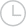2019-11-15 13:25:00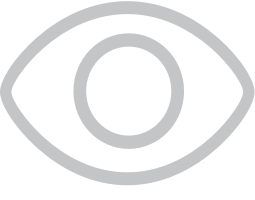5133

【精品问答】python技术1000问（2）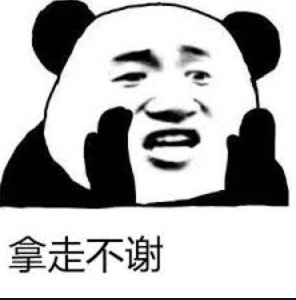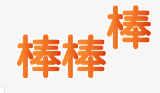python热门问题

Python基础测验 一、填空题

Python使用符号 # 标示单行注释；以 缩进对齐 划分语句块。

Python序列类型包括 字符串列表元组 三种； _字典_是Python中唯一的映射类型。

Python序列中的可变数据类型有列表字典，不可变数据类型有 字符串数组元组

Python的数字类型分为 整数长整数浮点型复数 等子类型。

1.下列哪个语句在Python中是非法的？ （ B

A、x = y = z = 1 B、x = (y = z + 1)

C、x, y = y, x D、x += y

2.关于Python内存管理，下列说法错误的是 （ B

A、变量不必事先声明

B、变量无须先创建和赋值而直接使用

C、变量无须指定类型

D、可以使用del释放资源

3.下面哪个不是Python合法的标识符 （ B

A、int32 B、40XL C、self

D、name 第一个字符必须是字母或下划线

4.下列表达式的值为True的是 （ C

A、(2**=3)2>2

C、1==1 and 2!=1 D、not(1==1 and 0!=1)

5.Python不支持的数据类型有 （ A

A、char B、int

C、float D、list

6.关于字符串下列说法错误的是 （B

A、字符应该视为长度为 1的字符串

B、字符串以标志字符串的结束

C、既可以用单引号，也可以用双引号创建字符串

D、在三引号字符串中可以包含换行回车等特殊字符

7、以下不能创建一个字典的语句是 （C

A、dict1 = {} B、dict2 = { 3 : 5 }

C、dict3 = {[1,2,3]: “uestc”}

D、dict4 = {(1,2,3): “uestc”}

8、下列Python语句正确的是 （D

A、min = x if x

B、max = x > y ? x : y

C、if (x > y)print x

D、while 1>2: print(“hello”）

**1.假设寄存器R1中存储的数值为10，执行完下面两条指令后，寄存器R2中存储的结果是什么？ **

mov R2,R1

2.请写出下面程序的运行结果。

``````a=10

b=30

def func(a,b)

a=a+b

return a

b=func(a,b)

print(a,b)

``````

3.读程序写结果。

``````L=[1,1.3,”2”,”China”,[“I”,”am”,”another”,”list”]]

L.append(“Hello World!”)

print(L)

print(L[0:4])

print(L[0:4:2])

print(L[-5:-2:-1])

print(L[-2:-5:-1])

``````

``````print(L) [1,1.3,?2?,?China?,[,I?,?am?,?another?,?list?],?Hello World!?]

print(L[0:4]) [1,1.3,?2?,?China?]

print(L[0:4:2]) [1,?2?]

print(L[0:5:-1]) []

print(L[-5:-2:-1]) []

print(L[-2:-5:-1]) [,china?,?2?,1.3]
``````

1.Python如何定义一个函数，并试写一个函数，给定n，返回n以内的斐波那契数列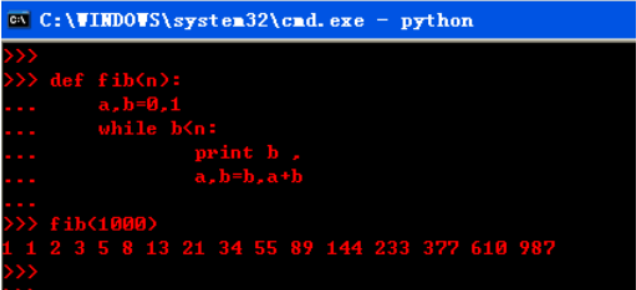2.什么是模块，模块是如何导入解释器的，分别有哪几种方法？并把上题中的斐波那契数列以模块方式导入，在解释器交互届面调入该函数。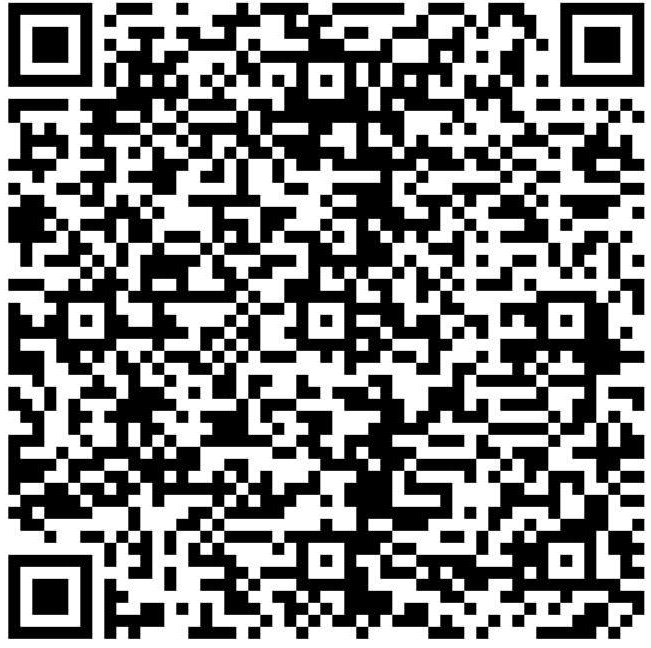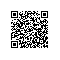取消 提交回答